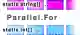# C# Parallel.For Examples

Use Parallel.For to call a method on multiple threads. Measure the performance improvement.

Parallel.For. A loop iterates over a method call many times. Sometimes, the method calls can be called in a parallel way—in any order.A speedup. When we can use parallel calls, we can speed up some programs by 4 times on a quad-core processor. We can use Parallel.For to make this optimization easier.

Parallel example. Using Parallel.For makes programs easier to parallelize. We can place each element in an input array, and then have a method write an output in another array.
And: We can call Parallel.For to call the method in parallel, giving us speedups of several times.
Tip: If each input and output position is separate, no conflicts will arise during execution, making parallel processing safe.
Note: I have used this style of code with Parallel.For (and Invoke) to speed up important programs (where validity is critical).
Parallel.InvokeForC# program that uses Parallel.For using System; using System.Threading.Tasks; class Program { static string[] _arrayToProcessInParallel = new string; static int[] _results = new int; static void ProcessArrayAtIndex(int index) { var element = _arrayToProcessInParallel; // Do something with array at this index. // ... Store in results array at this index. _results[index] = element.Length; } static void Main() { // Process array in parallel. // ... Each call is limited to its index in the arrays. // So no conflicts will arise. Parallel.For(0, _arrayToProcessInParallel.Length, i => ProcessArrayAtIndex(i)); // Access results array, all threads are done now. for (int i = 0; i < _results.Length; i++) { } Console.WriteLine("DONE"); } } Output DONE

Benchmark. Consider this program—we have a "values" array with 1000 integer elements. And our Example() method sums, and computes the product for, these elements.
And: The results are discarded. The Example method must be called many times—and these calls can be done in any order.
Version 1: We call Example in the Parallel.For method. Executions are performed on multiple threads.
Version 2: We run the Example method sequentially, on 1 thread. No multi-threading is done.
Result: The Parallel.For version finishes about 4 times faster. The computer being used has a quad-core processor.
C# program that uses Parallel.For using System; using System.Threading.Tasks; using System.Diagnostics; using System.Linq; class Program { static int[] _values = Enumerable.Range(0, 1000).ToArray(); static void Example(int x) { // Sum all the elements in the array. int sum = 0; int product = 1; foreach (var element in _values) { sum += element; product *= element; } } static void Main() { const int max = 10; const int inner = 100000; var s1 = Stopwatch.StartNew(); // Version 1: use Parallel.For. for (int i = 0; i < max; i++) { Parallel.For(0, inner, Example); } s1.Stop(); var s2 = Stopwatch.StartNew(); // Version 2: use for-loop. for (int i = 0; i < max; i++) { for (int z = 0; z < inner; z++) { Example(z); } } s2.Stop(); Console.WriteLine(((double)(s1.Elapsed.TotalMilliseconds * 1000000) / max).ToString("0.00 ns")); Console.WriteLine(((double)(s2.Elapsed.TotalMilliseconds * 1000000) / max).ToString("0.00 ns")); Console.Read(); } } Output 11229670.00 ns Parallel.For 46920150.00 ns for

Real-world usage. In my experience, Parallel.For is one of the safest and easiest ways to parallelize a program. As long as the threads only act on their own data, it is a good solution.

A summary. With Parallel.For we can do certain methods in parallel. This does not always help, but in tasks where the CPU usage is intensive, and no dependencies are present, it may help.

Home

© 2007-2020 sam allen. send bug reports to info@dotnetperls.com.